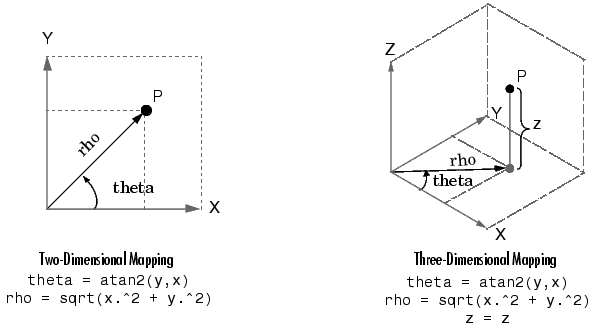MATLAB Function Referencecart2pol

Transform Cartesian coordinates to polar or cylindrical

Syntax

• ```[THETA,RHO,Z] = cart2pol(X,Y,Z)
[THETA,RHO] = cart2pol(X,Y)
```

Description

```[THETA,RHO,Z] = cart2pol(X,Y,Z) ``` transforms three-dimensional Cartesian coordinates stored in corresponding elements of arrays `X`, `Y`, and `Z`, into cylindrical coordinates. `THETA` is a counterclockwise angular displacement in radians from the positive x-axis, `RHO` is the distance from the origin to a point in the x-y plane, and `Z` is the height above the x-y plane. Arrays `X`, `Y`, and `Z` must be the same size (or any can be scalar).

```[THETA,RHO] = cart2pol(X,Y) ``` transforms two-dimensional Cartesian coordinates stored in corresponding elements of arrays `X` and `Y` into polar coordinates.

Algorithm

The mapping from two-dimensional Cartesian coordinates to polar coordinates, and from three-dimensional Cartesian coordinates to cylindrical coordinates isSee Also

`cart2sph`, `pol2cart`, `sph2cart`

© 1994-2005 The MathWorks, Inc.